# Particles

## Constituents of the Atom

Model of an atom

The key information for subatomic particles are shown in the table below.

Particle Charge Relative Mass

An atom normally has the same number of protons and electrons. This means that the overall charge of an atom is zero or neutral. This is because the charge from all of the protons combined ‘cancels out’ the overall charge from all of the electrons.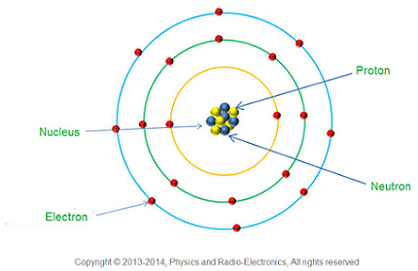For every type of matter particle we’ve found, there also exists a corresponding antimatter particle, or antiparticle.

Antiparticles look and behave just like their matter particle counterparts, except they have opposite charges. For instance, a proton is electrically positive whereas an antiproton is electrically negative. Gravity affects matter and antimatter the same way because gravity is not a charged property and a matter particle has the same mass as its antiparticle. When a matter particle and antimatter particle meet, they annihilate into pure energy! The anti-matter pair of an electron is called a positron.

Nuclide Notation

The constituents of atoms are shown in a particular way known as nuclide notation.

The general form is given below: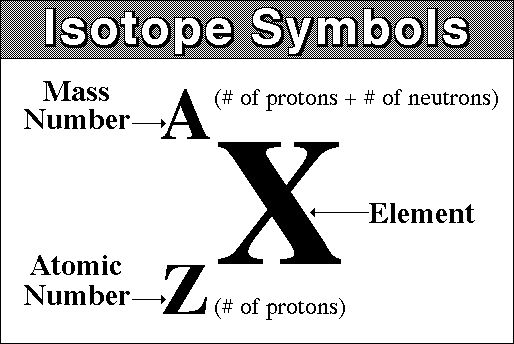As above, the mass number is both protons and neutrons and the atomic number is just protons.

Sometimes the atomic number is also called the proton number.

If you need to work out how many neutrons there are in an atom then you need to minus the atomic number from the mass number or:

Number of neutrons = A - Z

Let’s look at an example:This tells us that there are:

Isotopes

An isotope is an element that has a certain number of neutrons. This means that not all elements have the same number of neutrons, but they must have the same number of protons.

All atoms are isotopes.

Specific Charge

The specific charge of a particle is a ratio between the total charge and the total mass.

Specific Charge = ChargeMass

The units of specific charge is Ckg-1

What is the relative charge of a positron?
1
Explanation: +1 is correct!
A calcium ion is formed by removing two electrons from an atom of 4020Ca . What is the specific charge of the calcium ion?
Your answer should include: 4.8 / 106
Explanation: 4.8 × 106 C kg–1

## Stable & Unstable Nuclei

Strong nuclear force

There are four fundamental forces in nature. The strong nuclear force (also known as the strong force), the weak force, the electromagnetic force and gravity.

We will focus on the strong force for now and return to the others in another learning episode.

The strong force keeps the nucleus together and stable. It has a short range attraction up to 3 fm and a very short range repulsion closer than 0.5 fm.

Unstable nuclei

Sometimes the nucleus can become unstable. This can be for a few reasons such as having too much energy or being large and heavy. It might have too many neutrons or too few (though this is very unlikely).

The nucleus will release energy to become more stable and sometimes it will also release matter as well. These can take the form of either alpha radiation (helium nucleus) or beta radiation (an electron).

Decay equations

We will focus on the alpha and beta decay equations.

Alpha: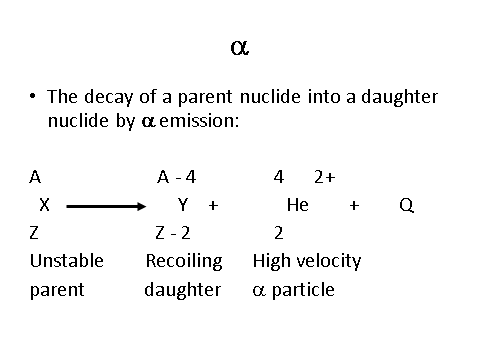Just like the alpha decay, beta decay also includes Q - the energy released in the decay.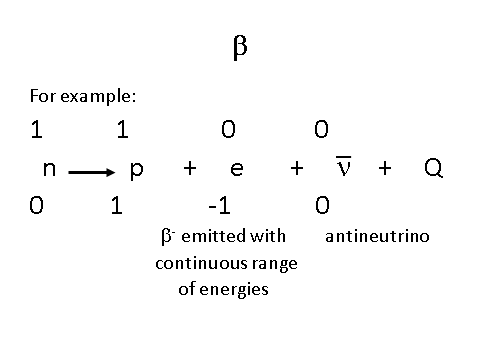It also has a new particle called an antineutrino

Neutrino

Neutrinos and antineutrinos were hypothesised due to the conservation law of momentum.

When beta decay occurs, there was some momentum that went missing and was unexplained. In order to ensure that momentum was conserved, scientists predicted that a new particle was also being created and ejected. They called this the neutrino.

## Particles Antiparticles & Photons

Anti-particles

As discussed beforehand all particles have an antiparticle pair.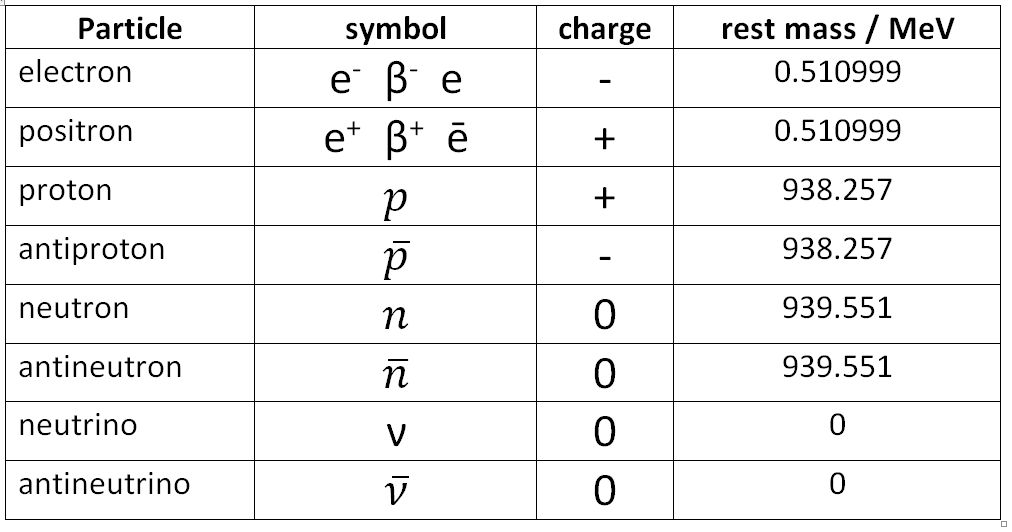Notice how all antiparticles have a line drawn on top the symbol. This shows that it is an antiparticle.

Photon Model of Electromagnetic Radiation

The energy of a photon can be calculated by the following relationship:

E = hf = hc

__E __= Energy (J)

h = Planck’s constant (m2 kgs-1)

__f __= Frequency (Hz)

c = Speed of light (ms-1)

= Wavelength (m)

Pair production and annihilation

As discussed all matter particles have antimatter pairs. When matter and antimatter collide they annihilate each other and produce two photons. This interaction conserves energy, momentum and charge.

For example, an electron and positron can annihilate each other to produce to photons.

This process is reversible, therefore a photon can produce a matter and an antimatter pair. This is called pair production.

## Particle Interactions

Four Fundamental Forces

The universe exists upon four forces and interactions. The example interactions include attraction, repulsion, decay and annihilation.

The four fundamental forces are:

Below is a table of their relative strengths and ranges.The particles in bold are NOT part of the course and will not be tested.

The bosons or force carrier particles is discussed in the next section below.

Exchange Particles

When forces interact between two objects, an exchange particle is passed between them.

When two objects are attracted to each other, we can imagine the exchange happening like this:__For repulsive interactions, the pass of the exchange particle can be modelled like this: __Weak interactions

Weak interactions are only limited to beta plus and beta minus decay, electron capture and electron-proton collisions. For these interactions the W+ and W- bosons are the exchange particles.

Feynman Diagrams

Feynman (a famous Physicist) came up with a simple way of showing these interactions. The diagrams have some set rules as shown below.

The first rule are the axis - Time is on the y and space is on the x. The length of the arrows to not represent any kind of scale unlike a normal graph axes.

Here is the list of all the ones you need to know: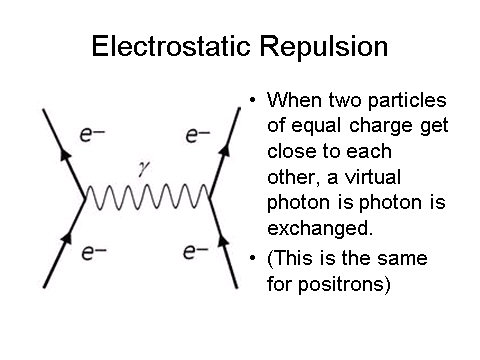Notice how all the particles are labelled! This is an easy few marks in an exam paper.

Which force is responsible for beta minus decay?
Weak
Explanation: Weak force is responsible for beta minus decay.
What is the exchange particle for the electromagnetic force?
Photon

## Classification of Particles

A hadron is a particle that is subject to the strong force.

All hadrons are made up of a combination of fundamental particles called quarks. There are six types of quarks which come in pairs.

The relative charges of the quarks are:

+⅔ = u, c, t

-⅓ = d, s, b

There are, of course, anti quarks. These have the opposing charges so an anti up has a charge of -⅔.

Hadrons are made up of two classes - Baryons and Mesons.

Baryons

A baryon is a particle that is made up of three quarks. The most commonly known baryons are protons and neutrons.

Their quark compositions are:

Each baryon also carries a ‘baryon number’. This is +1 for bayrons and -1 for antibaryons. If the particle is not a baryon then the number is a zero.

In all interactions the baryon number must be conserved. Energy and momentum are also conserved

The proton is the most stable baryon (as it is the lightest and has the least energy) and all bayrons will eventually decay into protons.

Mesons

A meson is made up of a quark and an antiquark. As such, mesons are not particularly stable particles and decay quickly. There are two mesons you are required to know; the pion and the kaon.

Pions

The structure of the different pions is shown below:

The pion occurs due to a strong interaction. When a proton and a neutron interact with each other, they exchange a pion.

Kaons

The structure of different kaons is shown below:

The kaon is a particle that can decay into a pion.

Strange particles

Strange particles are produced through the strong interaction and decay through the weak interaction.

Strangeness occurs if a particle has a strange quark and is there to reflect the fact that strange particles are always created in pairs.

Strangeness is conserved in strong interactions but not in weak interactions.

A strange quark has the number -1

An antistrange is +1

And if there is no strange quark then the particle has a strangeness of zero.

Leptons

Like before, there are also lepton numbers which must be conserved in interactions. However, there is an extra detail - each generation has its own leption number.

An e- has a number of +1 with an e+ having a number of -1, but this is a separate number of the muons and taus.

Leptons are not affected by the strong force.

When is strangeness conserved?
Your answer should include: Weak / Interactions
Explanation: Strangeness is conserved in weak interactions.
What is the quark composition of a proton?
udd
Is the reaction were a photon decays into an electron and an antimuon possible? Why?
Your answer should include: No / Conserved
Explanation: No, as the lepton number is not conserved.
Describe what happens to the quark combination when a neutron changes into a proton?
Your answer should include: Down / Up
Explanation: A down quark becomes an up quark.
In a strong interaction, what quantities are conserved?
Your answer should include: Energy / Momentum / Baryon Number / Strangeness